# Ray Optics: Reflection

Student Learning Objectives
Lessons / Lecture Notes
Important Equations
Example Problems
Applets and Animations

Student Learning Objectives

• To understand the ray model and how it applies to reflection.
• To understand and apply the law of reflection.
• To understand how, when, and why images are seen and apply these ideas to spherical mirrors.
• To use ray tracing to analyze image formation by mirrors.
• To use the mirror and magnification equations to analyze image formation by mirrors.

Lessons / Lecture Notes

The Physics Classroom (conceptual)

PY106 Notes from Boston University (algebra-based):

Physics 2C notes from Dr. Bobby W.S. Lau (algebra-based)

HyperPhysics (calculus-based)

Physics 4C notes from Dr. Bobby W.S. Lau (calculus-based)

Important Equations
(for algebra-based Physics)

Example Problems

Example Problems for algebra-based physics (from College Physics 2nd Edition by Knight, Jones, and Field):

Example Problems (Ray Optics)
Solutions to Example Problems (Ray Optics)

Example Problems for calculus-based physics (from Fundamentals of Physics 9th Edition by Halliday, Resnick, and Walker):

Example Problems (Images)
Solutions to Example Problems (Images)

Applets and Animations
 Reflection and Refraction of Light Waves (Huygen's Principle)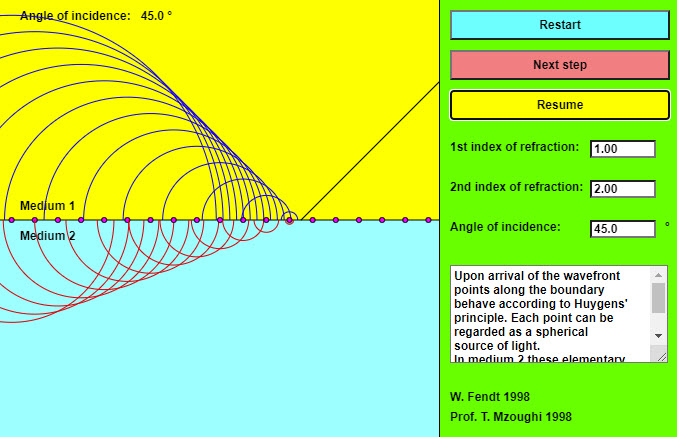This app is a sort of tutorial which explains the reflection and the refraction of waves by the principle of Huygens. Explanations of each of the steps are provided in the text box. Whenever a step is finished, press the "Next step" button! You can stop and continue the simulation by using the "Pause / Resume" button. The three text fields make it possible to vary the index of refraction of both media and the angle of incidence. The medium with the smaller index of refraction (the bigger phase velocity) is painted yellow, the other blue. Huygen's Principle - Reflection & Refraction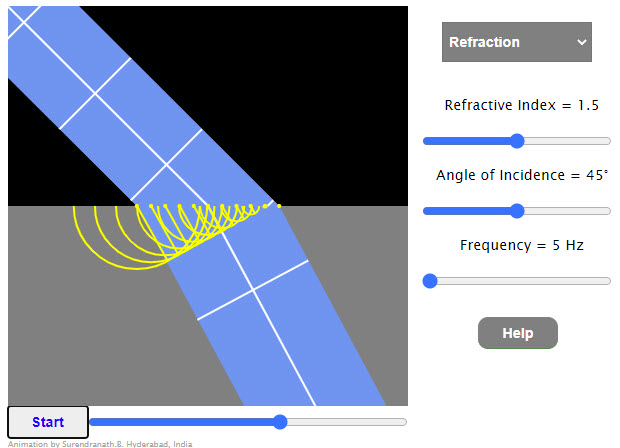Animation shows a plane wavefront being reflected or refracted at a plane boundary. The secondary wave front is shown as it is formed. Click/tap at the left edge to change the mode (single wavefront/multiple wavefronts) Drag at the boundary to change the width of the wavefront. Click/tap anywhere else to change the number of secondary sources. Reflection and Refraction of Light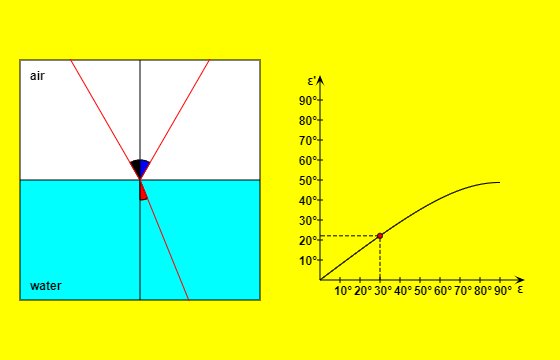A ray of light coming from the top left strikes the boundary surface of two media. (It is possible to choose the substances in both lists.) The medium which has the bigger index of refraction is painted blue, the other yellow. You can vary the incident ray with pressed mouse button. The app will show the reflected and the refracted ray and calculate the corresponding angles: Spherical Mirrors and Lenses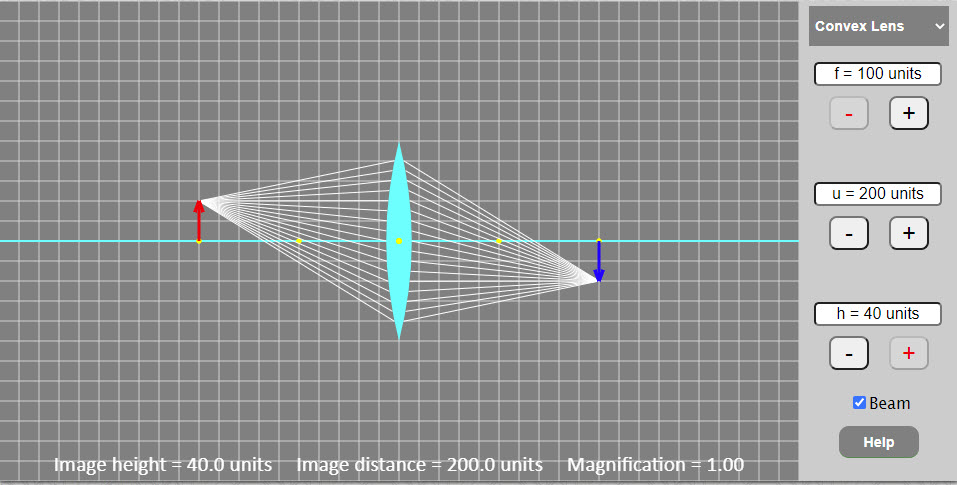A very nice applet showing the image formation by concave and convex mirrors and lenses. Geometric Optics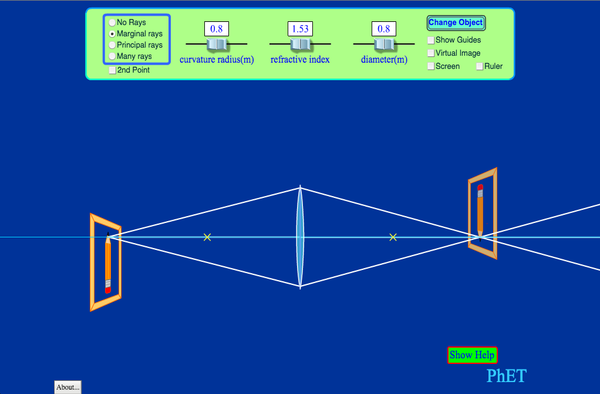How does a lens form an image? See how light rays are refracted by a lens. Watch how the image changes when you adjust the focal length of the lens, move the object, move the lens, or move the screen. Reflection from a Mirror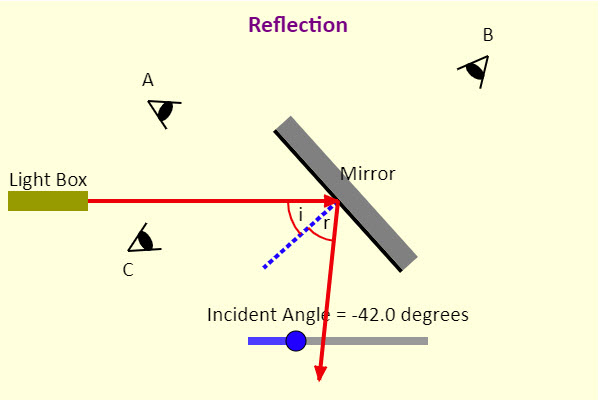Adjust the angle of the mirror and see what happens to the reflected beam! Determine the angle when each observer can see the light. Mirrors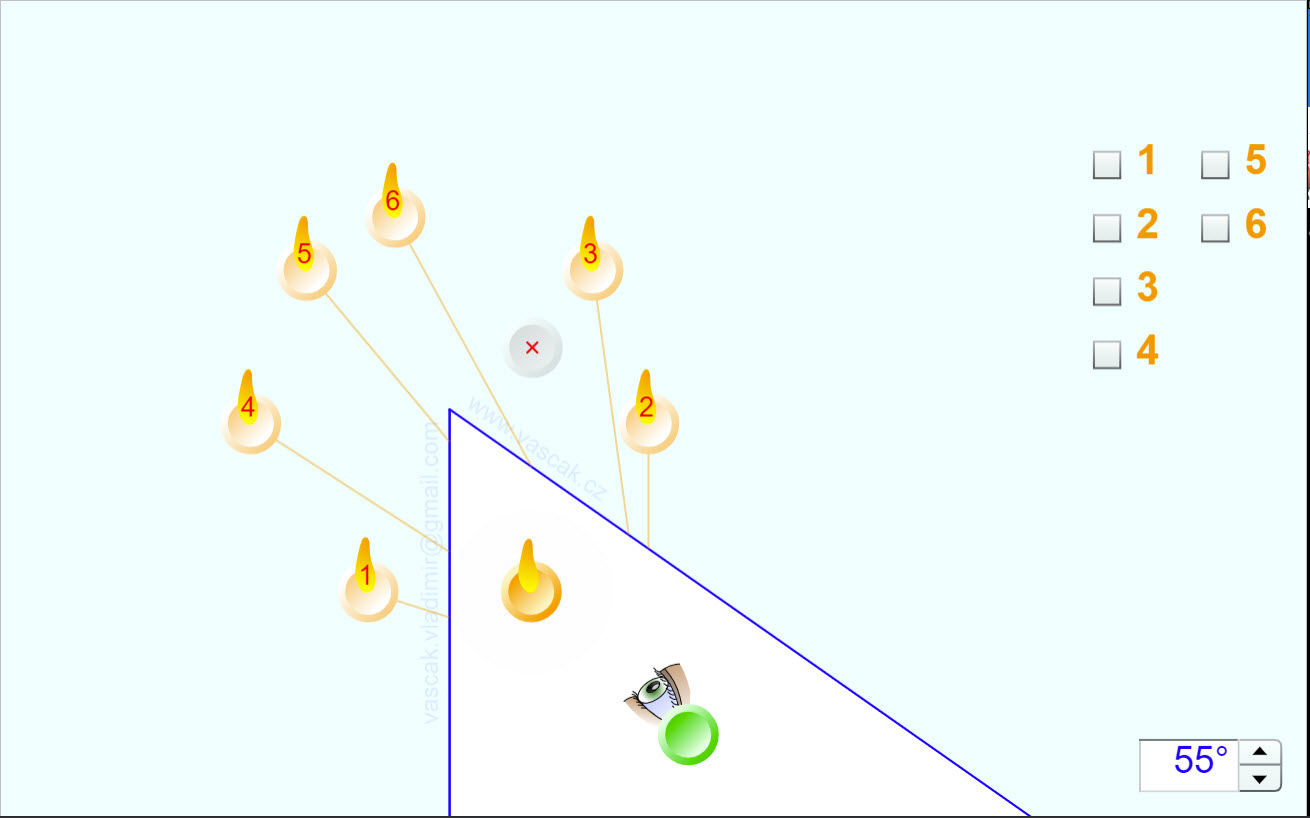A very cool applet showing the multiple images formed by a double mirror. The user can adjust the angle of the mirrors and see how the image locations change. Plane Mirror - How Tall?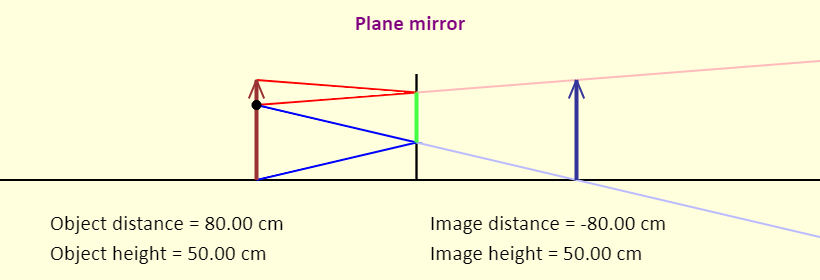What is the minimum height a mirror has to be for you to see your whole self in it? This simulation explores that concept. Note that the black dot on the object (red arrow) represents your eye. Lots of rays leave the top of your head, going in different directions, and reflect from the mirror. The one that reflects from the mirror and enters your eye is the one you use to see the image of the top of your head. This is shown in red. Similarly, lots of rays leave your foot, going in different directions, and reflect from the mirror. Again, the one that reflects from the mirror and enters your eye is the one you use to see the image of your foot. This is shown in blue. Exactly how tall does the mirror have to be for you to see your whole self? How should the mirror be positioned? Does the answer depend on how far you stand from the mirror? Mirrors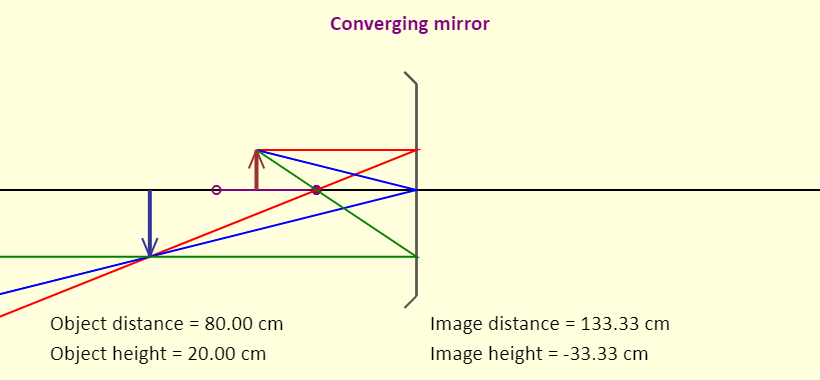An outstanding applet showing the image formation by converging, diverging, and plane mirrors. The applet shows the three principle rays for each diagram. Find the Focal Length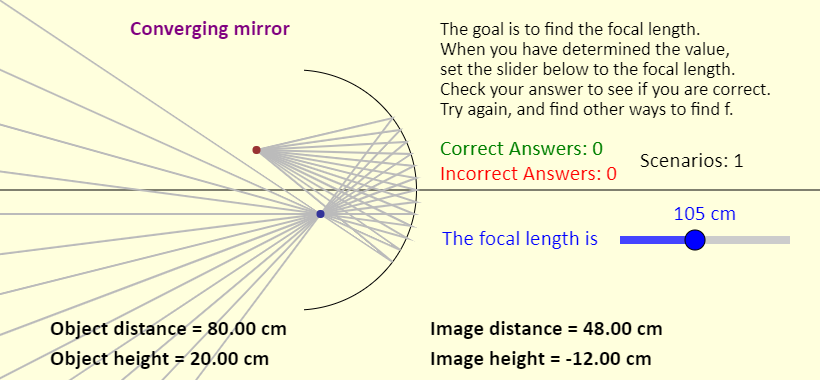The goal ultimately is to determine the focal length of a converging mirror. When you have figured it out, set the slider to that value, and then check your answer. See how many ways you can come up with to find the focal length. Concave Mirror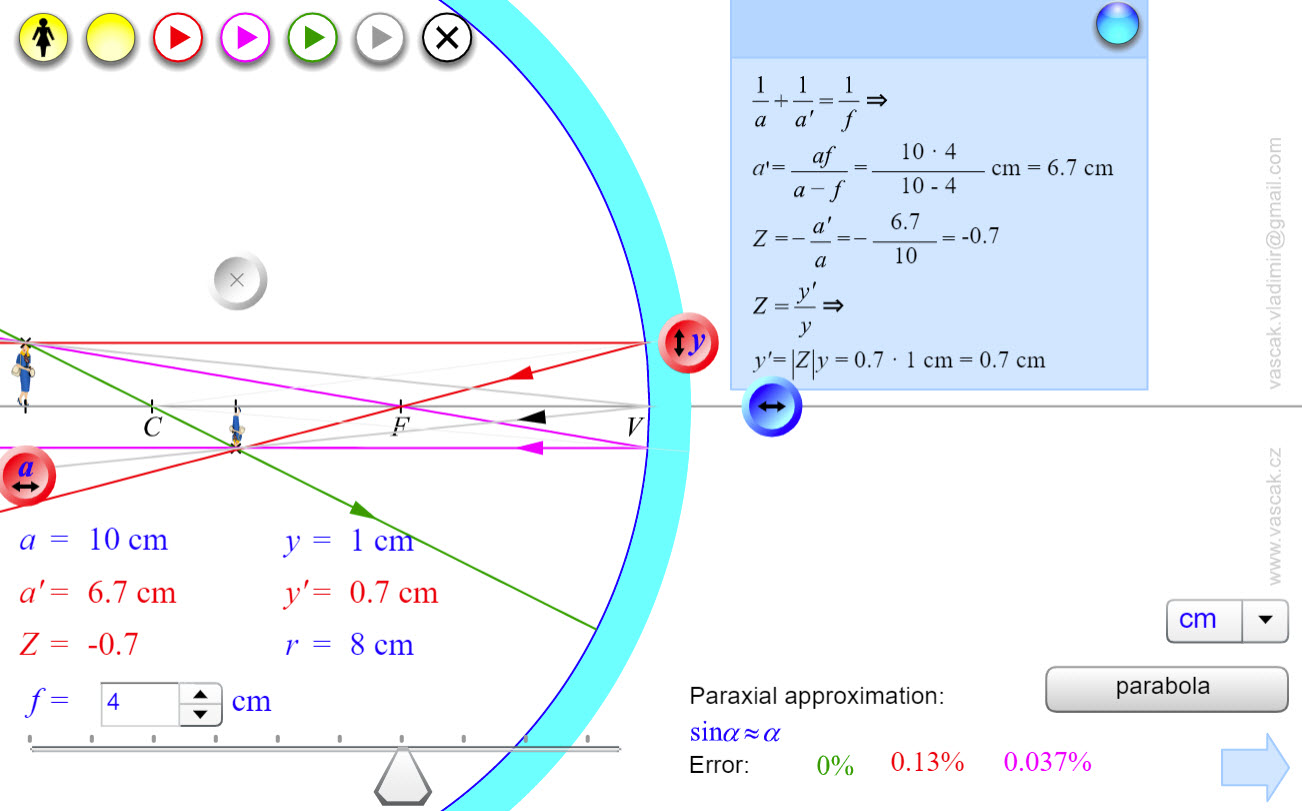An outstanding applet showing the image formation by a concave mirror and the four principle rays. Convex Mirror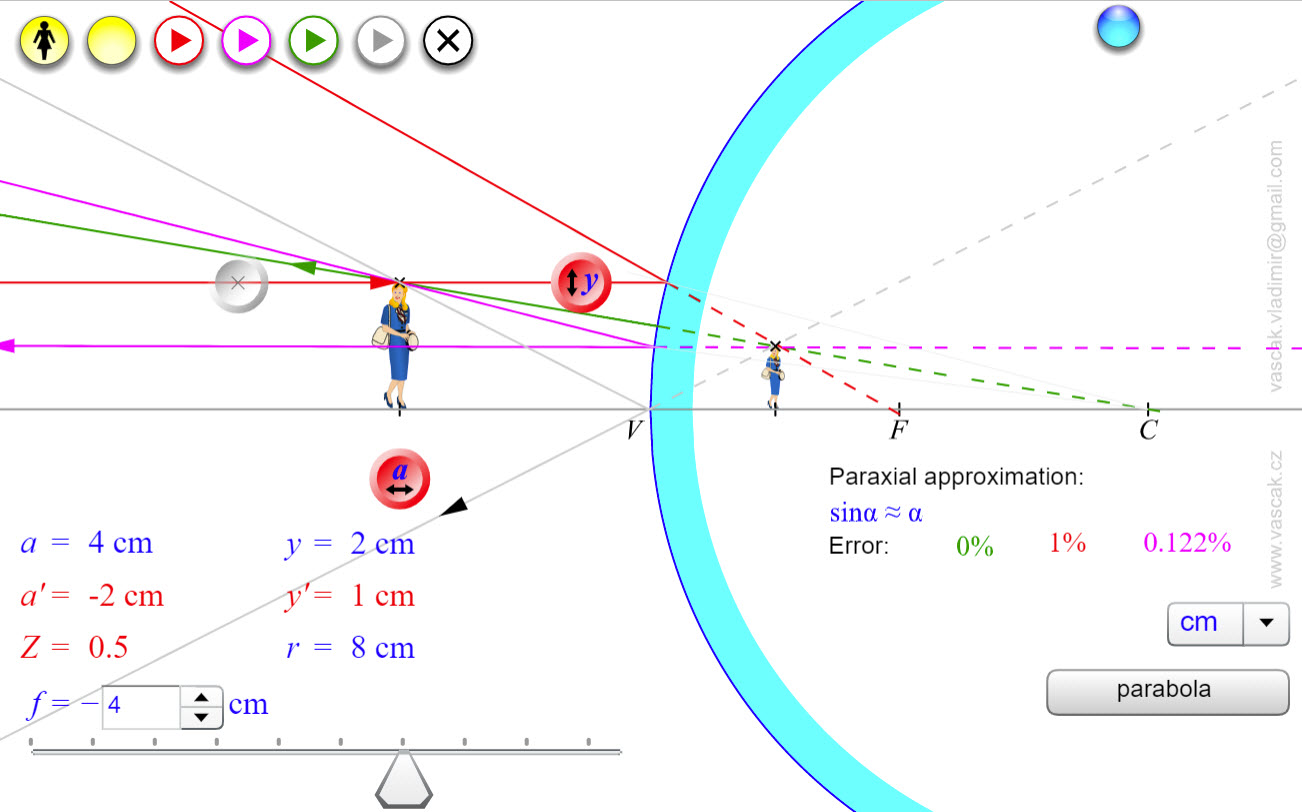An outstanding applet showing the image formation by a convex mirror and the four principle rays. What Kind of Mirror is Behind the Orange Curtain?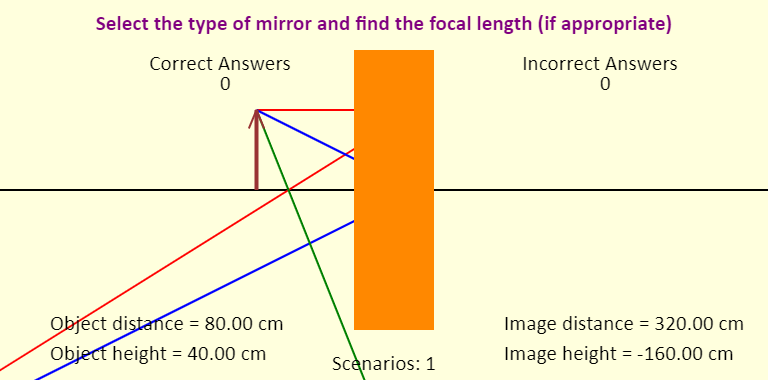Test your familiarity with mirrors. You can find the focal length of a converging or diverging mirror by calculating it, but see if you can get to the point where you can find it without doing a calculation. What is Behind the Orange Curtain?See if you can determine what is behind the curtain, and what its focal length is. What is Behind the Orange Curtain?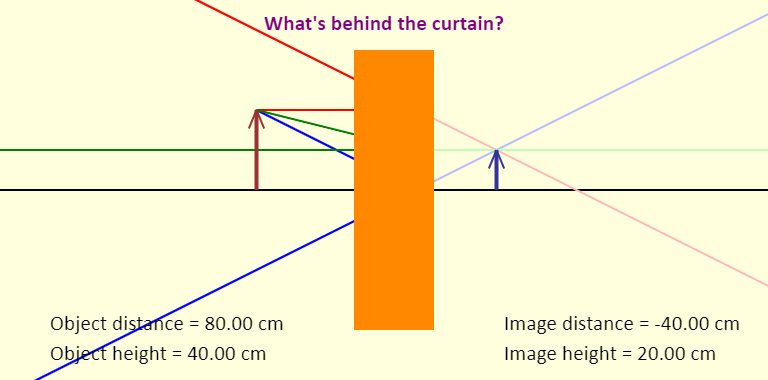See if you can determine what is behind the curtain, and what its focal length is. Spherical Aberration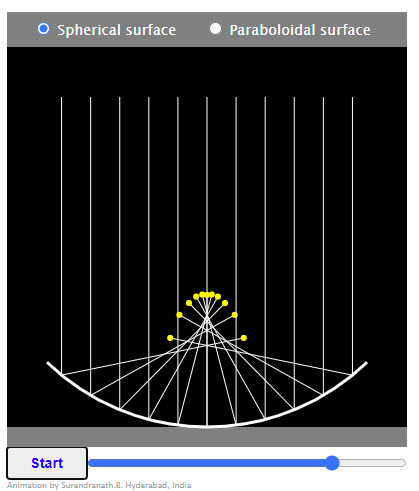Behaviour of a parallel beam on reflection from a Spherical/Paraboloidal concave surface is shown.